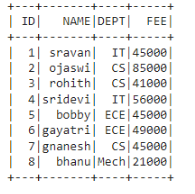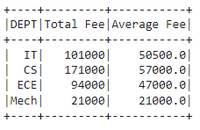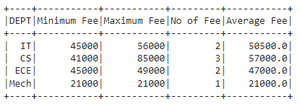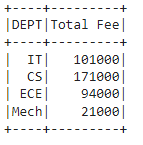GeeksforGeeks App
Open AppBrowser
Continue

# Renaming columns for PySpark DataFrames Aggregates

In this article, we will discuss how to rename columns for PySpark dataframe aggregates using Pyspark.

Dataframe in use:In PySpark,  groupBy() is used to collect the identical data into groups on the PySpark DataFrame and perform aggregate functions on the grouped data. These are available in functions module:

## Method 1: Using alias()

We can use this method to change the column name which is aggregated.

Syntax:

dataframe.groupBy(‘column_name_group’).agg(aggregate_function(‘column_name’).alias(“new_column_name”))

where,

• dataframe  is the input dataframe
• column_name_group is the grouped column
• aggregate_function is the function from the above functions
• column_name is the column where aggregation is performed
• new_column_name is the new name for column_name

Example 1: Aggregating DEPT column with sum() and avg() by changing FEE column name to Total Fee

## Python3

 `# importing module``import` `pyspark`` ` `# importing sparksession from pyspark.sql module``from` `pyspark.sql ``import` `SparkSession`` ` `#import functions``from` `pyspark.sql ``import` `functions`` ` `# creating sparksession and giving an app name``spark ``=` `SparkSession.builder.appName(``'sparkdf'``).getOrCreate()`` ` `# list  of student  data``data ``=` `[[``"1"``, ``"sravan"``, ``"IT"``, ``45000``],``        ``[``"2"``, ``"ojaswi"``, ``"CS"``, ``85000``],``        ``[``"3"``, ``"rohith"``, ``"CS"``, ``41000``],``        ``[``"4"``, ``"sridevi"``, ``"IT"``, ``56000``],``        ``[``"5"``, ``"bobby"``, ``"ECE"``, ``45000``],``        ``[``"6"``, ``"gayatri"``, ``"ECE"``, ``49000``],``        ``[``"7"``, ``"gnanesh"``, ``"CS"``, ``45000``],``        ``[``"8"``, ``"bhanu"``, ``"Mech"``, ``21000``]``        ``]`` ` `# specify column names``columns ``=` `[``'ID'``, ``'NAME'``, ``'DEPT'``, ``'FEE'``]`` ` `# creating a dataframe from the lists of data``dataframe ``=` `spark.createDataFrame(data, columns)`` ` ` ` `# aggregating DEPT column with sum() and avg() ``# by changing FEE column name to Total Fee``dataframe.groupBy(``'DEPT'``).agg(functions.``sum``(``'FEE'``).alias(``    ``"Total Fee"``), functions.avg(``'FEE'``).alias(``"Average Fee"``)).show()`

Output:Example 2 : Aggregating DEPT column with min(),count(),mean() and max() by changing FEE column name to Total Fee

## Python3

 `# importing module``import` `pyspark`` ` `# importing sparksession from pyspark.sql module``from` `pyspark.sql ``import` `SparkSession`` ` `#import functions``from` `pyspark.sql ``import` `functions`` ` `# creating sparksession and giving an app name``spark ``=` `SparkSession.builder.appName(``'sparkdf'``).getOrCreate()`` ` `# list  of student  data``data ``=` `[[``"1"``, ``"sravan"``, ``"IT"``, ``45000``],``        ``[``"2"``, ``"ojaswi"``, ``"CS"``, ``85000``],``        ``[``"3"``, ``"rohith"``, ``"CS"``, ``41000``],``        ``[``"4"``, ``"sridevi"``, ``"IT"``, ``56000``],``        ``[``"5"``, ``"bobby"``, ``"ECE"``, ``45000``],``        ``[``"6"``, ``"gayatri"``, ``"ECE"``, ``49000``],``        ``[``"7"``, ``"gnanesh"``, ``"CS"``, ``45000``],``        ``[``"8"``, ``"bhanu"``, ``"Mech"``, ``21000``]``        ``]`` ` `# specify column names``columns ``=` `[``'ID'``, ``'NAME'``, ``'DEPT'``, ``'FEE'``]`` ` `# creating a dataframe from the lists of data``dataframe ``=` `spark.createDataFrame(data, columns)`` ` ` ` `# aggregating DEPT column with min(),count(),mean() ``# and max() by changing FEE column name to Total Fee``dataframe.groupBy(``'DEPT'``).agg(functions.``min``(``'FEE'``).alias(``"Minimum Fee"``),``                              ``functions.``max``(``'FEE'``).alias(``"Maximum Fee"``), ``                              ``functions.count(``'FEE'``).alias(``"No of Fee"``),``                              ``functions.mean(``'FEE'``).alias(``"Average Fee"``)).show()`

Output:## Method 2: Using withColumnRenamed()

This takes a resultant aggregated column name and renames this column. After aggregation, It will return the column names as aggregate_operation(old_column)

so using this we can replace this with our new column

Syntax:

dataframe.groupBy(“column_name_group”).agg({“column_name”:”aggregate_operation”}).withColumnRenamed(“aggregate_operation(column_name)”, “new_column_name”)

Example: Aggregating DEPT column with sum() FEE and rename to Total Fee

## Python3

 `# importing module``import` `pyspark`` ` `# importing sparksession from pyspark.sql module``from` `pyspark.sql ``import` `SparkSession`` ` `#import functions``from` `pyspark.sql ``import` `functions`` ` `# creating sparksession and giving an app name``spark ``=` `SparkSession.builder.appName(``'sparkdf'``).getOrCreate()`` ` `# list  of student  data``data ``=` `[[``"1"``, ``"sravan"``, ``"IT"``, ``45000``],``        ``[``"2"``, ``"ojaswi"``, ``"CS"``, ``85000``],``        ``[``"3"``, ``"rohith"``, ``"CS"``, ``41000``],``        ``[``"4"``, ``"sridevi"``, ``"IT"``, ``56000``],``        ``[``"5"``, ``"bobby"``, ``"ECE"``, ``45000``],``        ``[``"6"``, ``"gayatri"``, ``"ECE"``, ``49000``],``        ``[``"7"``, ``"gnanesh"``, ``"CS"``, ``45000``],``        ``[``"8"``, ``"bhanu"``, ``"Mech"``, ``21000``]``        ``]`` ` `# specify column names``columns ``=` `[``'ID'``, ``'NAME'``, ``'DEPT'``, ``'FEE'``]`` ` `# creating a dataframe from the lists of data``dataframe ``=` `spark.createDataFrame(data, columns)`` ` ` ` `# aggregating DEPT column with sum() FEE and rename to Total Fee``dataframe.groupBy(``"DEPT"``).agg({``"FEE"``: ``"sum"``}).withColumnRenamed(``    ``"sum(FEE)"``, ``"Total Fee"``).show()`

Output:My Personal Notes arrow_drop_up